# Moments 1

## The turning effects of a force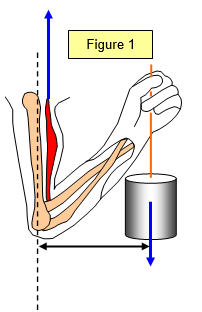Have you ever thought why it is much easier to open a door when you push on the edge furthest from the hinge or why objects seem much heavier when lifted with your arm outstretched? These effects are due to the turning effect of a force

If the line of action of a force does not pass through the centre of mass of the object then the force will exert a turning effect on the object and it will rotate (see Figure 2). The larger the force and the further the line of action from the centre of mass the greater the turning effect of the force will be.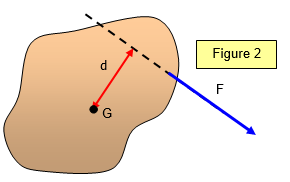The size of this turning effect is called the moment of the force and is defined as:

Moment of a force about a point = magnitude of force (F) x perpendicular distance (d) from the point to the line of action of the force.

Moment = Fd

Moments are measured in Newton metres (Nm), base units kgm2s-2.

One useful application of moments is the car wrench (a large spanner). When used to tighten up wheel nuts both the length of the wrench and the force to be used should be specified otherwise the nuts may be done up too tightly.

Problem
A boy pulls on a lever of length L = 0.5 m with a constant force of 20 N. Initially the lever makes an angle of 30o with the line of action of the force.

What is the moment of the force about the pivot when this angle is :
(a) 30o (b) 45o (c) 60o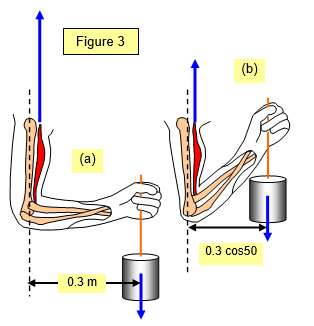Example problem
A 5 kg mass is held in a girls hand. What is the moment about her elbow if :
(a) she holds the mass as in Fig. 3(a) and
(b) she holds it as in Fig. 3(b)

(a) Moment = Fd = 5 x g x 0.3 = 15 Nm
(b) Moment = Fd = 5 x g x 0.3 x d = 5 x g x 0.3 cos 50 = 9.45 Nm

Notice the use of cos in (b) to find the perpendicular distance from the elbow to the line of action of the force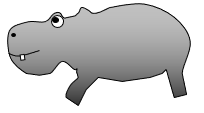Student investigation
Design a child's mobile.
It is to be made of three uniform rods (each 30 cm long and with a mass of 40 g). From each end of each rod must hang a toy animal, three monkeys (each with a mass of 20 g , two bears (each with a mass of 40g) and one hippo with mass of 60 g.

An interesting problem that combines both the resolution of a vector and the principle of moments is given in Figure 4.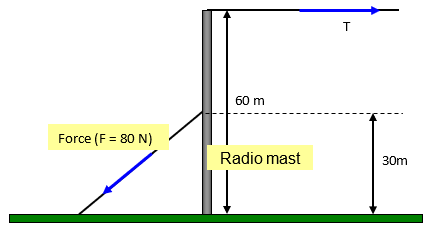A VERSION IN WORD IS AVAILABLE ON THE SCHOOLPHYSICS USB